# Bhattacharyya distance

Jump to: navigation, search

Several indices have been suggested in the statistical literature to reflect the degree of dissimilarity between any two probability distributions (cf. Probability distribution). Such indices have been variously called measures of distance between two distributions (see [a1], for instance), measures of separation (see [a2]), measures of discriminatory information [a3], [a4], and measures of variation-distance [a5]. While these indices have not all been introduced for exactly the same purpose, as the names given to them imply, they have the common property of increasing as the two distributions involved "move apart" . An index with this property may be called a measure of divergence of one distribution from another. A general method for generating measures of divergence has been discussed in [a6].

The Bhattacharyya distance is a measure of divergence. It can be defined formally as follows. Letbe a measure space, and letbe the set of all probability measures (cf. Probability measure) onthat are absolutely continuous with respect to. Consider two such probability measuresand let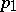andbe their respective density functions with respect to.

The Bhattacharyya coefficient betweenand, denoted by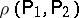, is defined by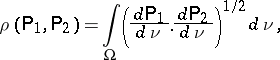whereis the Radon–Nikodým derivative (cf. Radon–Nikodým theorem) of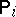() with respect to. It is also known as the Kakutani coefficient [a9] and the Matusita coefficient [a10]. Note thatdoes not depend on the measuredominatingand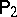.

It is easy to verify that

i)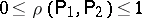;

ii)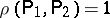if and only if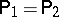;

iii)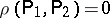if and only if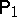is orthogonal to.

The Bhattacharyya distance between two probability distributionsand, denoted by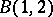, is defined by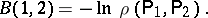Clearly,. The distancedoes not satisfy the triangle inequality (see [a7]). The Bhattacharyya distance comes out as a special case of the Chernoff distance (taking):The Hellinger distance [a8] between two probability measuresand, denoted by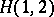, is related to the Bhattacharyya coefficient by the following relation: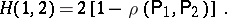is called the Bhattacharyya distance since it is defined through the Bhattacharyya coefficient. It should be noted that the distance defined in a statistical context by A. Bhattacharyya [a11] is different from.

The Bhattacharyya distance is successfully used in engineering and statistical sciences. In the context of control theory and in the study of the problem of signal selection [a7],is found superior to the Kullback–Leibler distance (cf. also Kullback–Leibler-type distance measures). If one uses the Bayes criterion for classification and attaches equal costs to each type of misclassification, then it has been shown [a12] that the total probability of misclassification is majorized by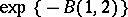. In the case of equal covariances, maximization ofyields the Fisher linear discriminant function. The Bhattacharyya distance is also used in evaluating the features in a two-class pattern recognition problem [a13]. Furthermore, it has been applied in time series discriminant analysis [a14], [a15], [a16], [a17].

See also [a18] and the references therein.